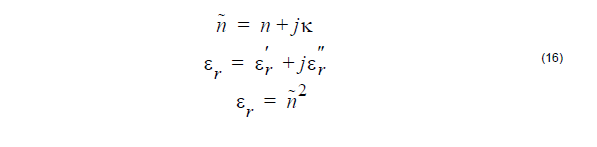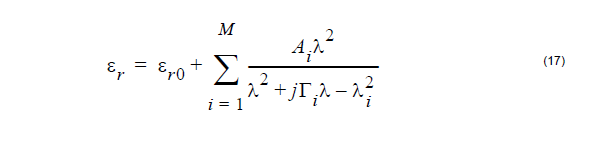Constant dielectric material is expressed by a complex refractive index value ( ) or
relative permittivity value (εr).Here, is the refractive index indicating the phase velocity informaiton in the
medium, while K is called the extinction coefficient, which indicates the amount of
absorption loss when the electromagnetic wave propagates through the material.
Note that the sign of the complex part is a matter of convention, which is important
due to possible confusion between loss and gain. From OptiFDTD version 9,
Engineering convention is used for the constant material definition, which means
negative imaginary part will bring the loss effect.

Sellmeier equation can be used for calculating the permittivity with the reference
wavelength (λ).Where Ai is the strength, Γi is the damping factor or collision wavelength. λi is the
oscillating wavelength.